# Monday 22.2.21

Welcome back to another fun packed term of learning. This term we will be learning about volcanoes, specifically Pompeii, this will be covered in Geography. We will move on to money, graphs and perimeter in Math’s and magnets and friction in Science. RE will involve looking at some religious festivals that occur at this time of year. We will continue to look at the language of learning and consider how we embody these.

Monday 22nd February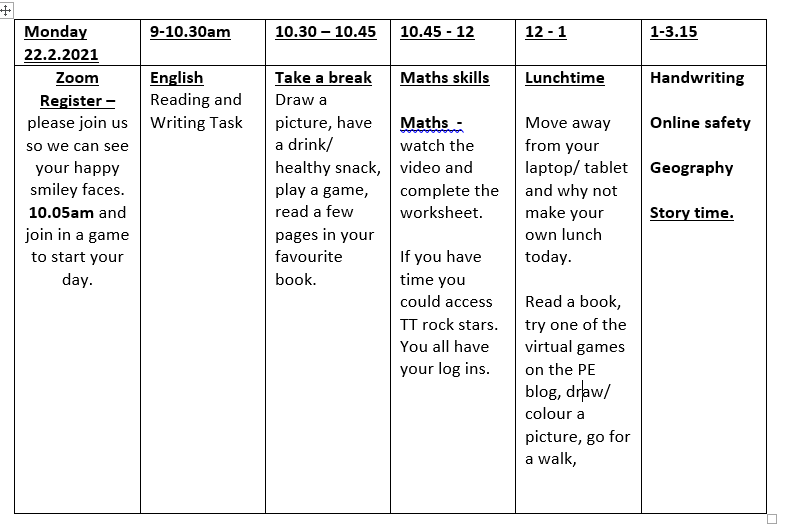Here is a ‘chopped up’ sentence. You should be familiar with this as we have done this a number of times in class. Look and read the words carefully and build the sentence. It would really help you if you can write out the words on paper and move them around to build a sentence. The clue is that the sentence starts with a fronted adverbial for where – remember there is a comma to show the end of the fronted adverbial. Keep reading your sentence to check makes sense. Remember you can stroll your sentence as you build to help you – like we do in class.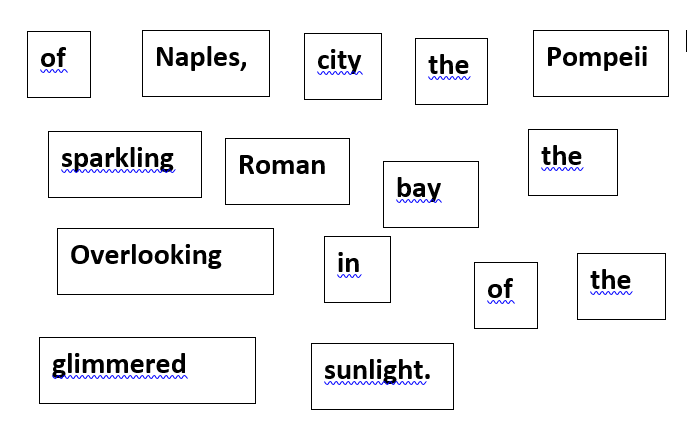Now, write down 5 things you know are facts (the truth from the sentence) and 5 inferences (things that might be true from what you have read in the sentence).

WRITING:

Now you have your sentence from your reading session  – there are two adjectives in the sentence used to describe nouns  – glimmered and sparkling  – can you think of similar adjectives you could use?

Also there is a fronted adverbial at the start of the sentence which describes where the story is set. Can you think of other fronted adverbials for where that would fit? The picture below could help you, you can use words like Near, Next to, By, In front of, In the background….. to help you.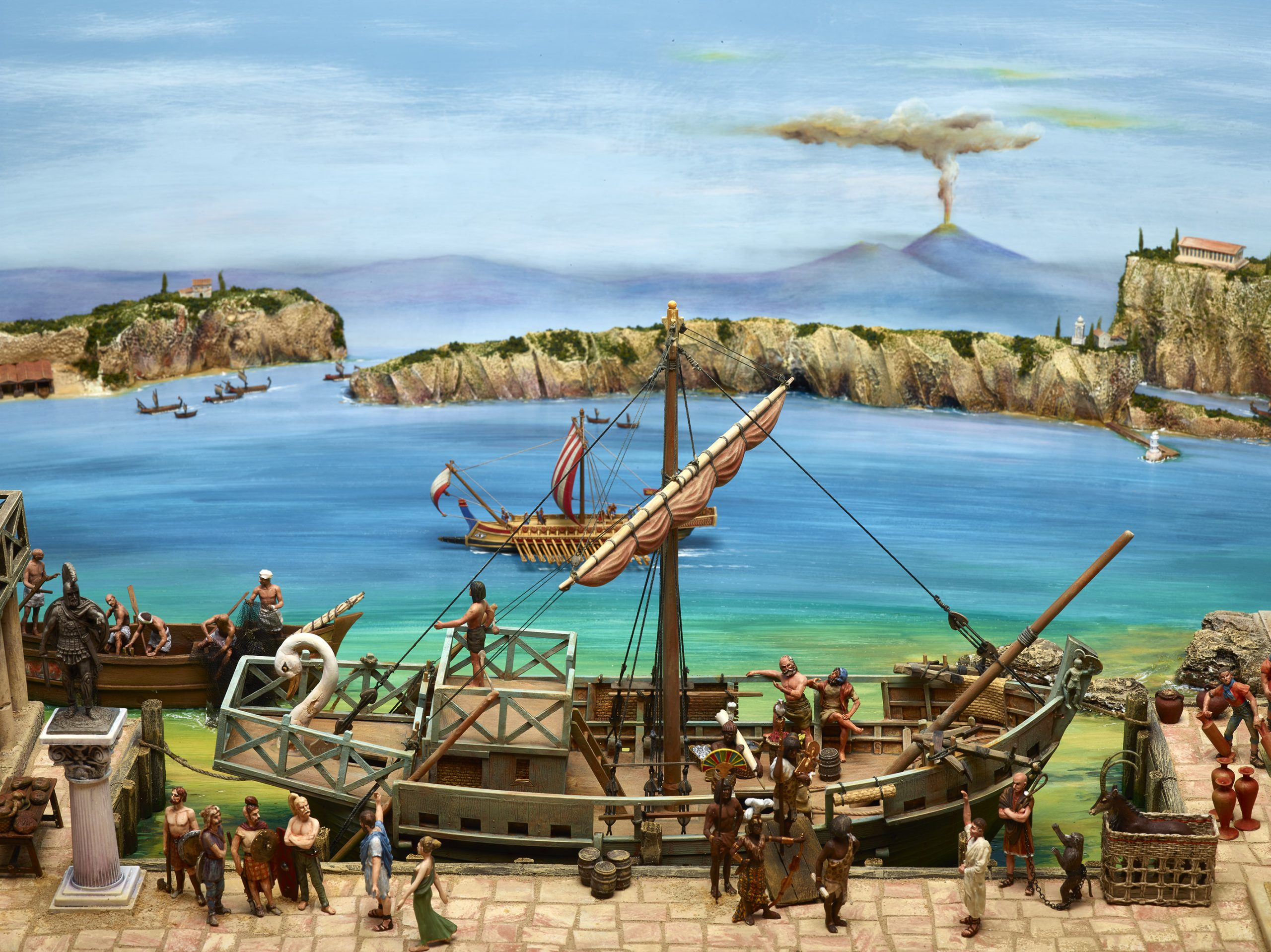Now use your sentence and write five sentences using different fronted adverbial (remember comma) and different adjectives for glimmering and sparkling for each sentence.

MATHS SKILLS: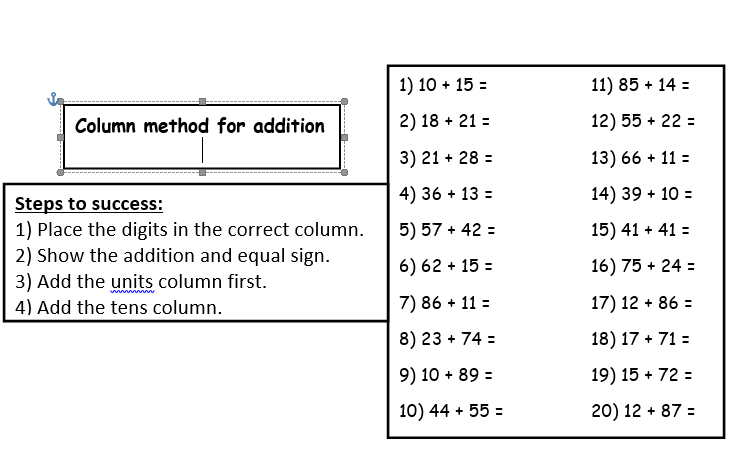MATHS:

HANDRWITING: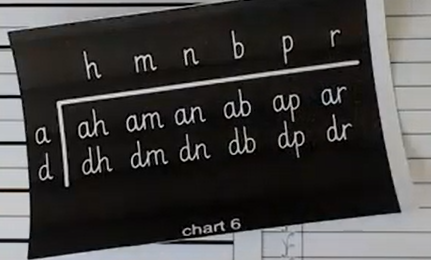It is important that you keep practicing your handwriting and the joins. It is good to not only improve your presentation but to get hands and fingers moving!

ONLINE SAFETY:

Watch the video about being kind online. Kindness is our value this week so it is important to be kind at home, in school and online.

It is important that you treat your friends the same online as you would if you saw them face to face.

Can you create your own Online Friendship Flower?  On each petal can you write a quality of what makes a good friend online?

Try and draw 6 petals. Be as creative as you like and send us a picture to our email address.

GEOGRAPHY:Geography- lesson 1 (final)

Mountains worksheet

STORY TIME https://youtu.be/hBRbFFRt3zY

### 41 thoughts on “Monday 22.2.21”

•22nd February 2021 at 8:42 am

ENGLISH
Overlooking in the sparkling city of Pomeii Napples in the bay of sunlight.

•22nd February 2021 at 11:30 am

Good attempt Alia, have another read of the sentence does it make sense?

Miss Brian

•22nd February 2021 at 8:57 am

Writing
In the blue sea

•22nd February 2021 at 11:30 am

You need to do five sentences please Alesha 🙂

Miss Brian

•22nd February 2021 at 9:01 am

1. 25
2. 49
3. 49
4. 49
5. 99
6. 77
7. 97
8. 97
9. 99
10. 99

•22nd February 2021 at 11:31 am

Good work Alia, have another look at the second question.

Miss Brian

•22nd February 2021 at 9:08 am

MATHS VIDEO
1. 2,4,6,8,10,12,14,16,18,20
2. 5,10,15,20,25,30,35,40,45,50
3. 6p
4.
5. They are different sizes

•22nd February 2021 at 11:38 am

Excellent work Alia 🙂

Miss Brian

•22nd February 2021 at 9:10 am

Maths
1.25
2.39
3.49
4.49
5.99
6.77
7.97
8.97
9.99
10.99

•22nd February 2021 at 11:33 am

Great job Alesha 🙂

Miss Brian

•22nd February 2021 at 9:19 am

Maths Video
1.2,4,6,8,10,12,14,16,18,20
5,10,15,20,25,30,35,40,45,50
6p

•22nd February 2021 at 10:33 am

English

Overlooking in the Naples, city the roman
sparkling Pompeii glimmered of the sunlight.

Maths Skills

10+15=25
18+21=39
21+28=49
36+13=49
57+42=99
67+15=77
86+11=97
23+74=97
10+89=99
44+55=99
85+14=99
55+22=77
66+11=77
39+10=49
41+41=82
75+24=99
12+86=98
17+71=88
15+72=87
12+87=99

•22nd February 2021 at 11:29 am

Have a read of you sentence does it make sense?
Great math work 🙂

Miss Brian

•22nd February 2021 at 1:31 pm

1. 25
2. 39
3. 49
4. 49
5. 99
6. 77
7. 97
8. 97
9. 99
10. 99
11 99
12. 88
13. 77
14. 49
15. 82
16. 99
17. 98
18. 88
19. 87
20. 99

•22nd February 2021 at 1:42 pm

Excellent work Denzel 🙂
Miss Brian

•22nd February 2021 at 10:51 am

In the city Naples, Roman sunlight over looking sparkling.
Maths;
1.25
2.46
3.49
4.49
5.99
6.77
7.97
8.97
9.99
10.99
11.99
12.77
13.77
14.49
15.82
16.99
17.98
18.88
19.87
20.89

•22nd February 2021 at 11:27 am

Have another look at your English work please Om, does the sentence you have built make sense?
Also have another look at questions 2, 18,19 and 20 for maths please.
You need to be completing all the English and Math sections of work please Om, not just the starters.

Miss Brian

•22nd February 2021 at 11:15 am

ONLINE SAFETY
Kind
Greatness
Incuregement
Loyalty
Friendly
Loveness

•22nd February 2021 at 11:36 am

Great words Alia, we spell encouragement and loveliness like this 🙂
Miss Brian

•22nd February 2021 at 11:16 am

Thoughtful
Lovely
thankful

•22nd February 2021 at 11:36 am

Are these your friendship flower petal qualities Alesha, if so great job 🙂

Miss Brian

•22nd February 2021 at 11:30 am

Maths skills
25
39
49
99
77
97
99
99
99
77
77
49
82
99
98
88
87
99

•22nd February 2021 at 11:42 am

in the roman city of Naples, Pompeii overlooking the sparkling bay of the glimmering sunlight

•22nd February 2021 at 11:45 am

Good attempt Cayden, have another look, Overlooking has a capital O do you think that means it should be the start of the sentence?
Miss Brian

•22nd February 2021 at 11:46 am

In the roman city of Naples, Pompeii overlooking the sparkling baby of the glimmering sunlight

•22nd February 2021 at 11:47 am

1) 25
2) 39
3) 49
4) 49
5) 99
6) 67
7) 97
8) 97
9) 99
10) 99
11) 99
12) 77
13) 77
14) 49
15) 82
16) 99
17) 98
18) 88
19) 87
20) 99

•22nd February 2021 at 1:03 pm

Very well done Kaya, have another look at question 6 🙂

Miss Brian

•22nd February 2021 at 12:25 pm

➗➕➖

Maths/Mathematics
1. 25|
2. 39|
3. 49|
4. 49|
5. 99|
6. 77|
7. 97|
8. 9734|
9. 99|
10. 991. 25|

➗➕➖
2. 49|
3. 49|
4. 49|
5. 99|
6. 77|
7. 98|
8. 97|
9. 99|
10. 100|
________

•22nd February 2021 at 1:04 pm

Good job Cayden!
Keep it up,
Miss Brian

•22nd February 2021 at 1:23 pm

10+15 = 25 10+15 = 25. 18+21 = 39. 21+28 = 49. 36+13 = 49. 57+42 = 99. 62+15 = 77. 86+11 = 97. 23+74 = 97 10+15 = 25. 18+21 = 39. 21+28 = 49. 36+13 = 49. 57+42 = 99. 62+15 = 77. 86+11 = 97. 23+74 = 97. 10+89 = 99. 44+55 = 99. 85+14 = 99. 55+22 = 77. 66+11 = 77. 39+10 = 49. 41+41 = 82. 75+24 = 99. 12+86 = 98. 17+71 = 88. 15+72 = 87. 12+87 = 99.

•22nd February 2021 at 1:41 pm

Great work Princess, keep it up 🙂
Miss Brian

•22nd February 2021 at 2:12 pm

10+15 = 25. 18+21 = 49. 21+28 =49. 36+13 = 49. 57+15 = 99. 62+15 = 77. 86+11 = 97. 23+74=97. 10+89 = 99. 44+55 = 99. 85+14 = 99. 55+22 = 77. 66+11 = 77. 39+10 = 49. 41+41 = 82. 75+24 = 99. 12+86 = 98. 17+71 = 88. 15+72 = 87. 12+87 =99. Welcome back year three

•22nd February 2021 at 3:11 pm

Great work Priscilla, welcome back to you too!

Miss Brian

•22nd February 2021 at 3:08 pm

Overlooking the bay of Naples, the Roman city of Pompei glimmered in the sparking sunlight.

Next to the bay of Naples, the Roman city of Pompei shined in the warm sunlight.

By the bay of Naples, the Roman city of pompei
shining in the hot sunlight.

in front of Naples, the Roman city of pompei
Sparklecl in the Bright sunlight

•22nd February 2021 at 3:12 pm

Fantastic work Callan! You are a star 🙂
Miss Brian

•22nd February 2021 at 6:39 pm

Geography

Summit –
The highest point of the mountain

Face – the visable side of the mountain

Snowline – above this line snow covers the mountain

Ridge – long narrow top connecting mountains

The slope – an incline or decline on side of mountain

•22nd February 2021 at 4:55 pm

Maths

2 4 6 8 10 12 14 16 18 20

5 10 15 20 25 30 35 40 45 50

6

102

1p 2p 5p 10p 20p 50p

£1 £2 £5

£10 £20 £50

5p
10p
20p
2p

20p+20p+10p=50p
2p+2p+2+5p=11p
there are 61p
50p+11p=61p

one on the right becaues 20+20=40
10+10+5+5+5+2+2=39
worksheet
1.
20+2+50+1+10+5=88
2.
there are 7p
there are 50p
there are 20p
there are 12p
there are 60p
so there are £1.37p
3.
there are 23p
there are 28p
there are 62p
so there are
£1.13
4.
23+72=95p
5.
6p>3p
50p>40p
15<20
10=10
6.
=74
=70
yes because she has 4p more
7.
yes becaues she has 96p
chengling

1.10+15=25
2.18+21=39
3.21+28=49
4.36+13=49
5.57+42=99
6.62+15=79
7.86+11=96
8.23+74=97
9.10+89=99
10.44+55=99
11.85+14=99
12.55+22=77
13.66+11=77
14.39+10=49
15.41+41=82
16.75+24=99
17.12+86=98
18.17+71=82
19.15+72=87
20.12+87=99

•22nd February 2021 at 6:02 pm

Math wotk sheet

1.
1p
2p
5p
10p
20p
50p

2.
a. 7p
b.50p
c. 20p
d.12p
e.60p

3
a.23p
b.28p
c.63p

4
85p

5.
a.
d. =

6. Annie is incorrect because she has 74p and tommy has 90p

7. Yes as she has 96p

•22nd February 2021 at 6:23 pm

English –

Naples, in Roman city of Pompeii.
Overlooking of the bay glimmered the sparkling sunlight.

•22nd February 2021 at 7:17 pm
•